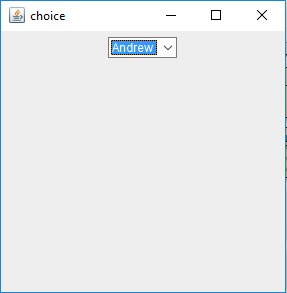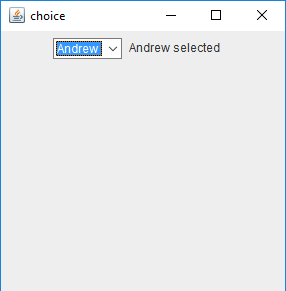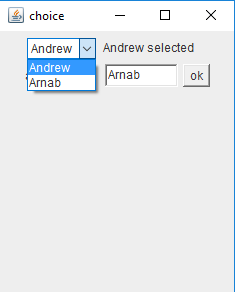Open In App

# Java AWT | Choice Class

Choice class is part of Java Abstract Window Toolkit(AWT). The Choice class presents a pop- up menu for the user, the user may select an item from the popup menu. The selected item appears on the top. The Choice class inherits the Component.

Constructor for the Choice class

• Choice() : creates an new empty choice menu .

#### Different Methods for the Choice Class

MethodExplanation
getAccessibleContext()gets the AccessibleContext associated with this Choice.
getItem(int i)gets the string at the specified index in this Choice menu
getItemCount()returns the number of items in this Choice menu.
getItemListeners()returns an array of all the item listeners registered on this choice.
getListeners(Class l)returns an array of all the objects currently registered as FooListeners upon this Choice.
getSelectedIndex()returns the index of the currently selected item.
getSelectedItem()gets a representation of the current choice as a string.
insert(String s, int i)inserts the item into this choice at the specified position.
paramString()returns a string representing the state of this Choice menu.
processEvent(AWTEvent e)processes events on this choice.
processItemEvent(ItemEvent e)processes item events occurring on this Choice menu by dispatching them to any registered ItemListener objects.
remove(int p)removes an item from the choice menu at the specified position.
remove(String s)removes the first occurrence of item from the Choice menu.
removeAll()removes all items from the choice menu.
select(int p)sets the selected item in this Choice menu to be the item at the specified position.

Below programs illustrate the Choice class in Java AWT:

• Program to create a simple choice and add elements to it:

 `// Java Program to create a simple ``// choice and add elements to it .``import` `java.awt.*;``import` `javax.swing.*;`` ` `class` `choice {`` ` `    ``// choice``    ``static` `Choice c;`` ` `    ``// frame``    ``static` `JFrame f;`` ` `    ``// default constructor``    ``choice()``    ``{``    ``}`` ` `    ``// Main Method``    ``public` `static` `void` `main(String args[])``    ``{`` ` `        ``// create a frame``        ``f = ``new` `JFrame(``"choice"``);`` ` `        ``// create e panel``        ``JPanel p = ``new` `JPanel();`` ` `        ``// create a choice``        ``c = ``new` `Choice();`` ` `        ``// add element to the list``        ``c.add(``"Andrew"``);``        ``c.add(``"Arnab"``);``        ``c.add(``"Ankit"``);`` ` `        ``// add choice to panel``        ``p.add(c);`` ` `        ``// add panel to the frame``        ``f.add(p);`` ` `        ``// show the frame``        ``f.show();``        ``f.setSize(``300``, ``300``);``    ``}``}`

Output :• Program to create a simple choice and add ItemListener to it:

 `// Java  Program to create a simple``// choice and add ItemListener to it``import` `java.awt.*;``import` `javax.swing.*;``import` `java.awt.event.*;`` ` `class` `choice ``implements` `ItemListener {`` ` `    ``// choice``    ``static` `Choice c;`` ` `    ``// frame``    ``static` `JFrame f;`` ` `    ``// label``    ``static` `Label l;`` ` `    ``// default constructor``    ``choice()``    ``{``    ``}`` ` `    ``// Main Method``    ``public` `static` `void` `main(String args[])``    ``{`` ` `        ``// create a frame``        ``f = ``new` `JFrame(``"choice"``);`` ` `        ``// object``        ``choice ch = ``new` `choice();`` ` `        ``// create e panel``        ``JPanel p = ``new` `JPanel();`` ` `        ``// create a choice``        ``c = ``new` `Choice();`` ` `        ``// add element to the list``        ``c.add(``"Andrew"``);``        ``c.add(``"Arnab"``);``        ``c.add(``"Ankit"``);`` ` `        ``// add itemListener to it``        ``c.addItemListener(ch);`` ` `        ``// create a label``        ``l = ``new` `Label();`` ` `        ``// set the label text``        ``l.setText(c.getSelectedItem() + ``" selected"``);`` ` `        ``// add choice to panel``        ``p.add(c);``        ``p.add(l);`` ` `        ``// add panel to the frame``        ``f.add(p);`` ` `        ``// show the frame``        ``f.show();``        ``f.setSize(``300``, ``300``);``    ``}`` ` `    ``// if an item is selected``    ``public` `void` `itemStateChanged(ItemEvent e)``    ``{``        ``l.setText(c.getSelectedItem() + ``" selected"``);``    ``}``}`

Output :• Program to create a choice and manually add elements to it (using add(String s) function):

 `// Java Program to create a choice and``// manually add elements to it ``// (using add(String s) function)``import` `java.awt.*;``import` `javax.swing.*;``import` `java.awt.event.*;`` ` `class` `choice ``implements` `ItemListener, ActionListener {`` ` `    ``// choice``    ``static` `Choice c;`` ` `    ``// frame``    ``static` `JFrame f;`` ` `    ``// label``    ``static` `Label l;`` ` `    ``// textfield``    ``static` `TextField tf;`` ` `    ``// default constructor``    ``choice()``    ``{``    ``}`` ` `    ``// Main Method``    ``public` `static` `void` `main(String args[])``    ``{`` ` `        ``// create a frame``        ``f = ``new` `JFrame(``"choice"``);`` ` `        ``// object``        ``choice ch = ``new` `choice();`` ` `        ``// create e panel``        ``JPanel p = ``new` `JPanel();`` ` `        ``// create a choice``        ``c = ``new` `Choice();`` ` `        ``// add element to the list``        ``c.add(``"Andrew"``);`` ` `        ``// create a textfield``        ``tf = ``new` `TextField(``7``);`` ` `        ``// create a button``        ``Button b = ``new` `Button(``"ok"``);`` ` `        ``// add actionListener``        ``b.addActionListener(ch);`` ` `        ``// add itemListener to it``        ``c.addItemListener(ch);`` ` `        ``// create a label``        ``l = ``new` `Label();``        ``Label l1 = ``new` `Label(``"add names"``);`` ` `        ``// set the label text``        ``l.setText(c.getSelectedItem() + ``" selected"``);`` ` `        ``// add choice to panel``        ``p.add(c);``        ``p.add(l);``        ``p.add(l1);``        ``p.add(tf);``        ``p.add(b);`` ` `        ``// add panel to the frame``        ``f.add(p);`` ` `        ``// show the frame``        ``f.show();``        ``f.setSize(``250``, ``300``);``    ``}`` ` `    ``// if an item is selected``    ``public` `void` `itemStateChanged(ItemEvent e)``    ``{``        ``l.setText(c.getSelectedItem() + ``" selected"``);``    ``}`` ` `    ``// if button is pressed``    ``public` `void` `actionPerformed(ActionEvent e)``    ``{``        ``// add item to the choice``        ``c.add(tf.getText());``    ``}``}`

Output :Note: The programs might not run in an online IDE please use an offline IDE.